## Excel 中的字符计数：单元格和范围（简单指南）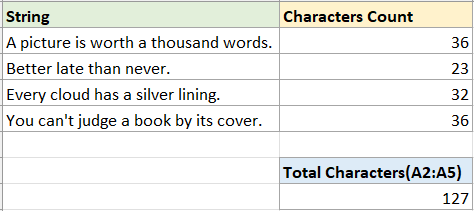### 字符数

#### 计算单个单元格中的字符数

##### 步骤 1：选择一个空白单元格并使用 LEN 函数

``=LEN(A2)``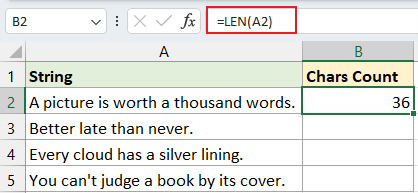##### 步骤 2（可选）：将自动填充手柄拖动到要计算字符数的单元格上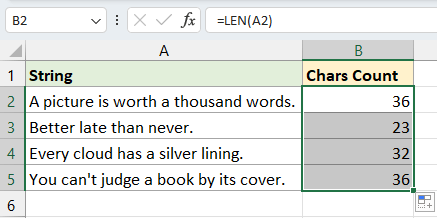#### 计算单元格区域中的字符数

``=SUMPRODUCT(LEN(A2:A5))``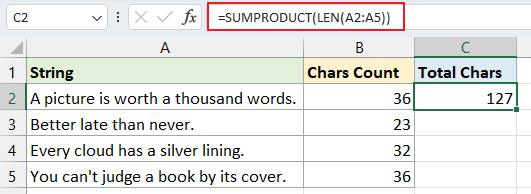:
• 您可以使用以下公式来计算范围 A2:A5 中的字符总数。
`` =SUM(LEN(A2:A5))``
但是，如果使用的是 Excel 2019 之前的版本，请确保按 Shift + Ctrl + Enter 同时获得准确的结果。
• 如果您需要计算几个不连续单元格（例如单元格 A2 和 A5）中的字符总数，则上述公式将无法正常工作。 请改用以下公式：
``=SUM(LEN(A2),LEN(A5))``

### 某些特定字符很重要

#### 计算单元格或范围内区分大小写的特定字符

##### 方法 1：使用公式组合 LEN 和 SUBSTITUTE 函数
• 计算单元格中区分大小写的特定字符

例如，要计算单元格 A2 中字符“s”的数量，请使用以下公式并按 Enter 键：

``=LEN(A2)-LEN(SUBSTITUTE(A2,"s",""))``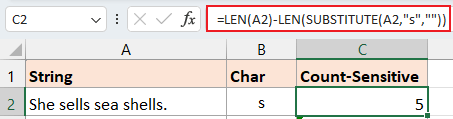公式解释：
• LEN(A2)：计算单元格 A2 中的字符总数。
• SUBSTITUTE(A2,"s","")：将所有字符“s”替换为空。
• LEN(SUBSTITUTE(A2,"s","")):获取A2中不包含字符“s”的字符长度。
• LEN(A2)-LEN(SUBSTITUTE(A2,"s",""))：单元格 A2 中的总字符数减去 A2 中不包含字符“s”的字符长度。 结果将是 A2 中字符“s”的数量。
备注：您可以使用单元格引用来指定公式中的字符，当您使用自动填充手柄填充公式时，该字符会自动调整。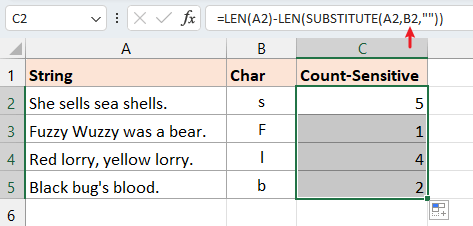• 计算一定范围内区分大小写的特定字符

如果您想计算 A2:A5 范围内的字符“s”，请使用以下公式并按 Enter 键：

``=SUMPRODUCT(LEN(A2:A5) - LEN(SUBSTITUTE(A2:A5,"s", "")))``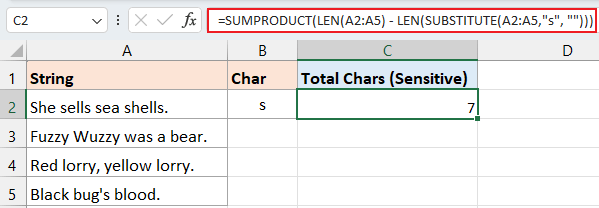##### 方法2：使用 Kutools for Excel 通过点击

1. 点击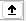，在 inside_text 文本框来选择要在其中计算特定字符的单元格。
2. 点击，在 查找文本 文本框来选择引用要计数的特定字符的单元格。 （或者在 Find_text 文本框中键入特定字符。）然后单击 OK.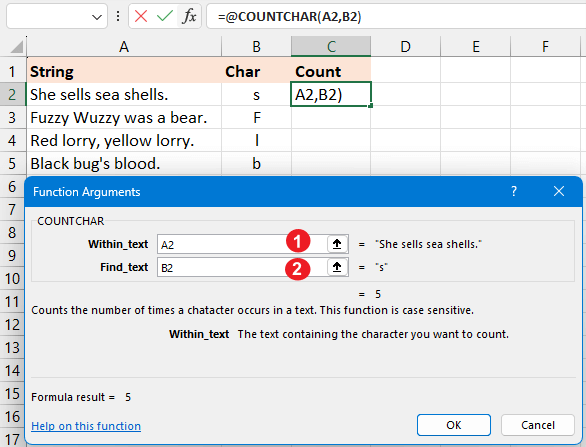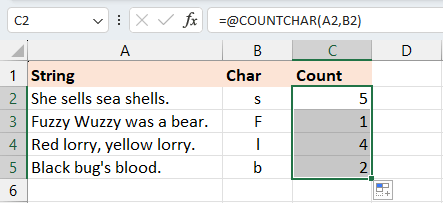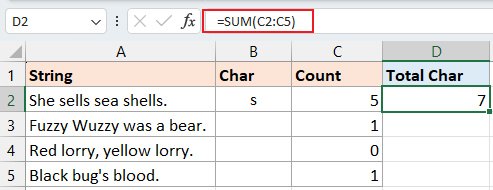#### 计算单元格或区域中不区分大小写的特定字符

• 计算单元格中不区分大小写的特定字符

如果要计算单元格 A2 中的字符“s”或“S”，可以使用以下公式并按 Enter 键：

``=LEN(A2) - LEN(SUBSTITUTE(UPPER(A2), UPPER("s"),""))``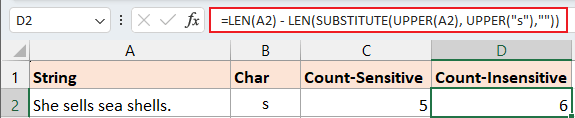公式解释：
• LEN(A2)：计算单元格 A2 中的字符总数。
• UPPER(“s”)：将“s”更改为“S”。
• UPPER(A2)：将单元格 A2 中的所有字母更改为大写。
• SUBSTITUTE(UPPER(A2), UPPER("s"),"")：将所有字符“S”替换为空。
• LEN(SUBSTITUTE(UPPER(A2), UPPER("s"),""))：获取A2中不含字符“s”和“S”的字符长度。
• LEN(A2)-LEN(SUBSTITUTE(A2,"s",""))：单元格 A2 中的总字符数减去 A2 中不包含字符“s”和“S”的字符长度。 结果将是 A2 中字符“s”和“S”的数量。
备注：您可以使用单元格引用来指定公式中的字符，当您使用自动填充手柄填充公式时，该字符会自动调整。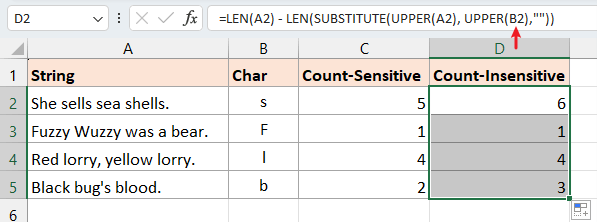• 计算范围内不区分大小写的特定字符

如果您想计算 A2:A5 范围内字符“s”和“S”的总数，请使用以下公式并按 Enter 键：

``=SUMPRODUCT(LEN(A2:A5) - LEN(SUBSTITUTE(UPPER(A2:A5), UPPER("s"), "")))``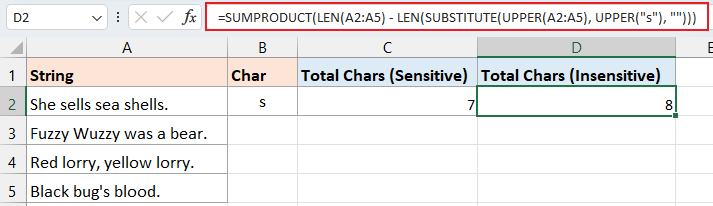### 计算某些文本的数量

#### 计算单元格或区域中区分大小写的某些文本

##### 方法 1：使用公式组合 LEN 和 SUBSTITUTE 函数
• 计算单元格中区分大小写的某些单词

例如，要计算单元格 A2 中单词“see”的数量，请使用以下公式并按 Enter 键：

``=(LEN(A2) - LEN(SUBSTITUTE(A2, "see", ""))) / LEN("see")``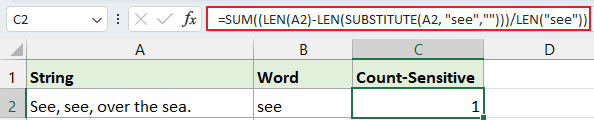备注：您可以使用单元格引用来指定公式中的特定文本，当您使用自动填充手柄填充公式时，该文本会自动调整。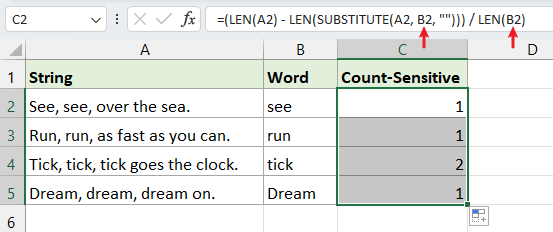• 计算一定范围内区分大小写的某些单词

如果您想计算范围 A2:A5 中的单词“and”，请使用以下公式并按 Enter 键：

``=SUMPRODUCT((LEN(A2:A5) - LEN(SUBSTITUTE(A2:A5, "and", ""))) / LEN("and"))``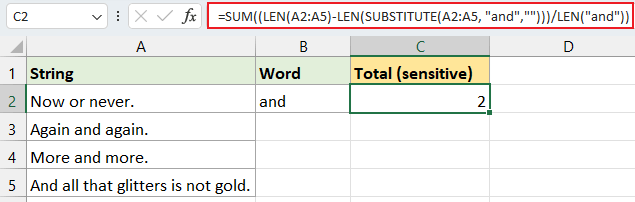##### 方法2：使用 Kutools for Excel 通过点击

• 点击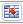，在 文本 文本框来选择要在其中计算特定单词的单元格。
• 点击，在 Word 文本框来选择引用要计数的特定单词的单元格。 （或在 Word 文本框中键入特定单词。）然后单击 OK.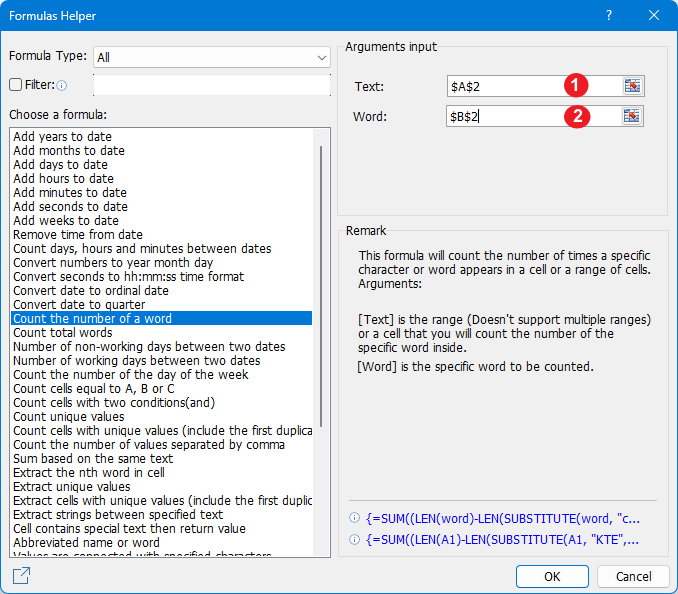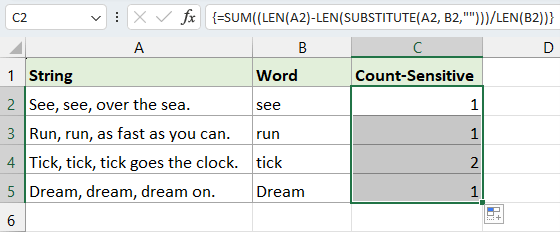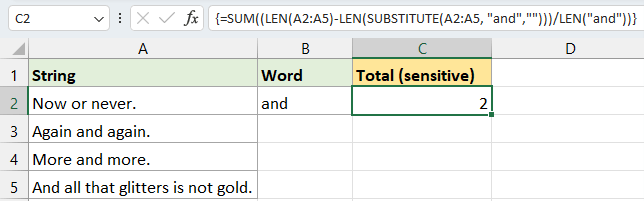#### 计算单元格或范围内不区分大小写的某些文本

• 计算单元格中不区分大小写的某些文本

要统计单元格 A2 中单词“see”的出现次数而不考虑大小写区别（无论是“SEE”、“see”、“SeE”等），您可以使用以下公式。 输入后，只需按 Enter 键：

``=(LEN(A2)-LEN(SUBSTITUTE(UPPER(A2), UPPER("see"),""))) / LEN("see")``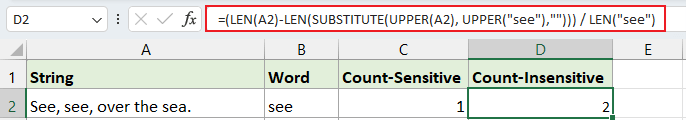备注：您可以使用单元格引用来指定公式中的单词，当您使用自动填充手柄填充公式时，该单词会自动调整。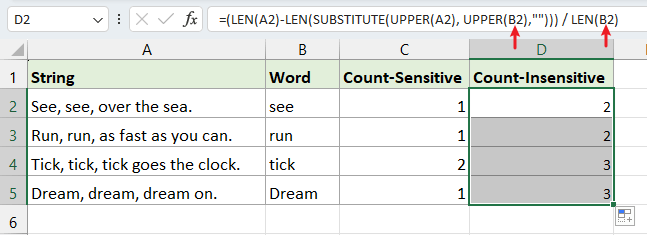• 计算范围内不区分大小写的特定字符

如果您想计算A2:A5范围内不区分大小写的单词“and”的总数，请使用下面的公式并按 Enter 键：

``=SUMPRODUCT((LEN(A2:A5) - LEN(SUBSTITUTE(UPPER(A2:A5), UPPER("and"), ""))) / LEN(UPPER("and")))``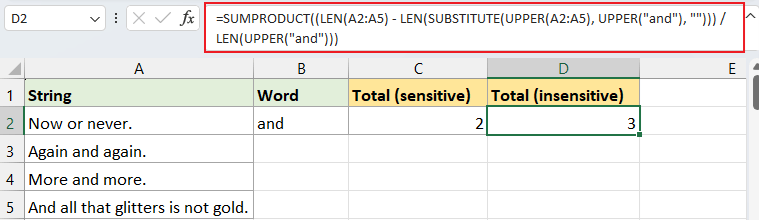### 最佳办公效率工具

#### Kutools for Excel - 助您脱颖而出

 热门特色: 查找、突出显示或识别重复项  |  删除空白行  |  合并列或单元格而不丢失数据  |  不使用公式进行四舍五入 ... 超级VLookup: 多重标准  |  多重价值  |  跨多页  |  模糊查询... 副词。 下拉列表: 简易下拉列表  |  依赖下拉列表  |  多选下拉列表... 列管理器: 添加特定数量的列  |  移动列  |  切换隐藏列的可见性状态  |  将列与 选择相同和不同的单元格 ... 特色功能: 网格焦点  |  设计图  |  大方程式酒吧  |  工作簿和工作表管理器 | 资源库 （自动文本）  |  日期选择器  |  合并工作表  |  加密/解密单元格  |  按列表发送电子邮件  |  超级筛选  |  特殊过滤器 （过滤粗体/斜体/删除线...）... 前 15 个工具集:  12 文本 工具 (添加文本, 删除字符 ...）  |  50+ 图表 类型 (甘特图 ...）  |  40+ 实用 公式 (根据生日计算年龄 ...）  |  19 插入 工具 (插入二维码, 从路径插入图片 ...）  |  12 转化 工具 (小写金额转大写, 货币兑换 ...）  |  7 合并与拆分 工具 (高级组合行, 拆分 Excel 单元格 ...）  |  ... 和更多

Kutools for Excel 拥有 300 多项功能， 确保只需点击一下即可获得您所需要的...#### Office Tab - 在 Microsoft Office（包括 Excel）中启用分页式阅读和编辑

• 一秒钟即可在数十个打开的文档之间切换！
• 每天为您减少数百次鼠标单击，告别鼠标手。
• 查看和编辑多个文档时，可将您的工作效率提高50％。
• 为 Office（包括 Excel）带来高效的选项卡，就像 Chrome、Edge 和 Firefox 一样。No ratings yet. Be the first to rate!
This comment was minimized by the moderator on the site
To count all characters in a worksheet: - Rather than bother copying to Word, just use formula
' =SUMPRODUCT(LEN(A1:B5)) '.
It works, try it!!
In fact SUM(LEN(A1:B5)) will probably crash with #VALUE! error if used over large ranges, or a lot of characters are in each cell,
particularly in older versions of Excel like Excel2000.

So if you are getting #VALUE! errors when using ' SUM(LEN(A1:B5)) ', switch to SUMPRODUCT.
I prefer this to tediously copying to Word, because with SUMPRODUCT :-

1) It works over large ranges easily, on cell formating of any kind

2) You can deduct parts of a range that may not be relevant (e.g. a cell, row or column that contains personal notes not relevant to the data you want to count)
e.g. =SUMPRODUCT(LEN(B1:L5799)) - LEN(C4) removes the count of characters in Cell C4 from the whole range B1:L5799
=SUMPRODUCT(LEN(B1:L5799)) - SUMPRODUCT(LEN(B462:L462)) removes the count of characters in Row 462 from the whole range B1:L5799
=SUMPRODUCT(LEN(B1:L5799)) - SUMPRODUCT(LEN(D1:D5799)) removes the count of characters in Col. D from the whole range B1:L5799

3) You can add ranges on more than one sheet, so without pasting every sheet to Word, you can get a total no. of characters in the whole Workbook
e.g =SUMPRODUCT(LEN(B3:L799))+SUMPRODUCT(LEN(Sheet2!B4:C7))+SUMPRODUCT(LEN(Sheet3!A1:C7000)) is totaling the count for data on 3 sheets

4) These formulae will update as your worksheet progresses - otherwise you will have to keep pasting to Word every time you alter your data and need a revised count of the characters

5) It keeps your information in one place.

Warning A) - Both ' SUMPRODUCT(LEN(A1:B5)) ' and ' SUM(LEN(A1:B5)) ' will fail if any of the individual cells in the range contain a formula that cannot compute and produce an error value such as #VALUE!, #NULL!, #REF!, #NUM!,#DIV/0! etc. Always best to combine error protection to your formulae in every cell.
You can use Error protection on SUMPRODUCT to flag an error, such as ' =IF(ISERROR(SUMPRODUCT(LEN(B4:C7))),"err",SUMPRODUCT(LEN(B4:C7)))'
which outputs the message "err" if any cell contains an error, but you cannot obtain the character count of the range until the cell(s) causing the errors are corrected, or the range is modified to omit the cell(s) containing the error.
This comment was minimized by the moderator on the site
NAOMBA NISAIDIE KUJUA IDADI YA ROW NA COLUM KWENYE EXCEL
This comment was minimized by the moderator on the site